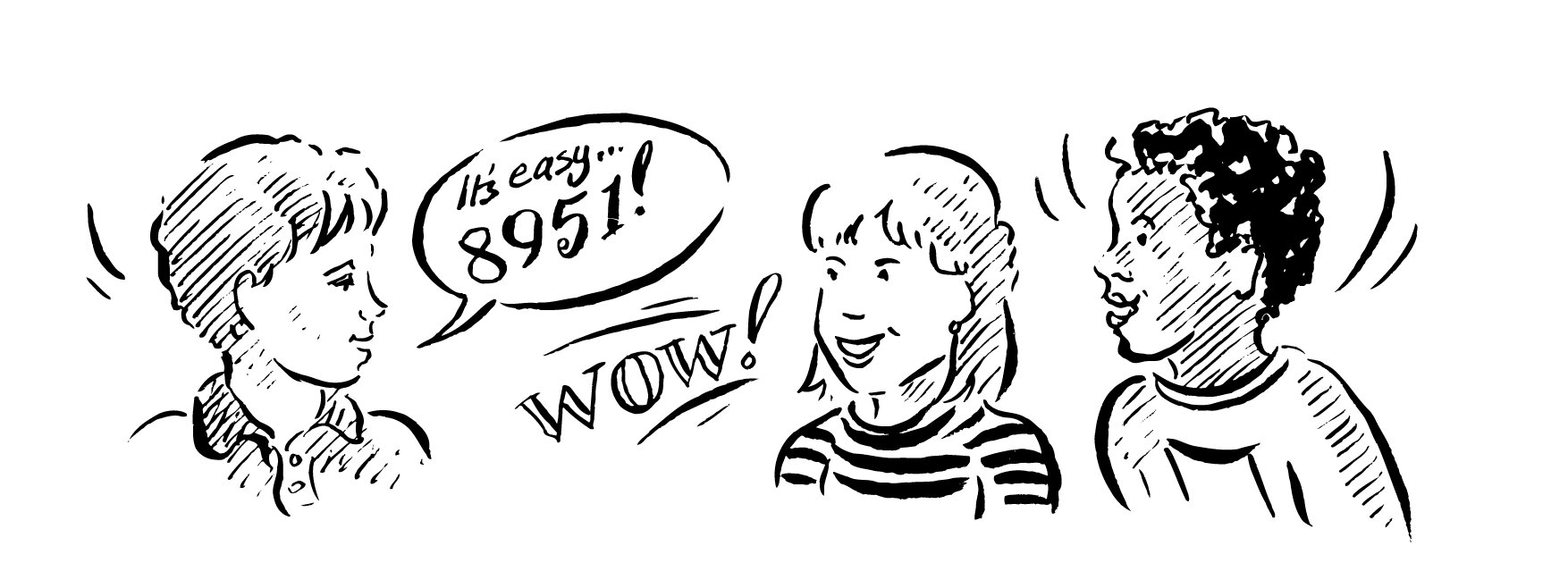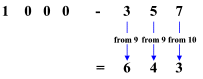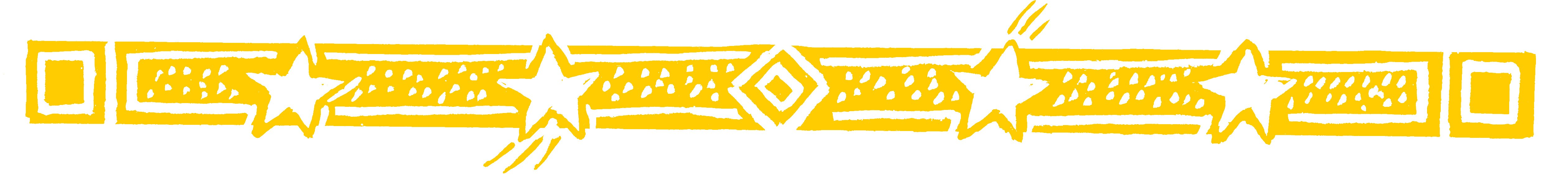Use the formula ALL FROM 9 AND THE LAST FROM 10 to perform instant subtractions.

• For example 1000 - 357 = 643

We simply take each figure in 357 from 9 and the last figure from 10.So the answer is 1000 - 357 = 643

And thats all there is to it!

This always works for subtractions from numbers consisting of a 1 followed by noughts: 100; 1000; 10,000 etc.

• Similarly 10,000 - 1049 = 8951• For 1000 - 83, in which we have more zeros than figures in the numbers being subtracted, we simply suppose 83 is 083.

So 1000 - 83 becomes 1000 - 083 = 917

Question Comment !
 Tutorial Title Question Title No Questions 0 Questions Asked 0 Skipped Questions 0 Correctly Answered 0 Wrongly Answered 0 Total Question Attempts 0 Current Question Attempts 0

Skip Question

Reset Test

Halt Test

 No Questions 0 Questions Asked 0 Skipped Questions 0 Correctly Answered 0 Wrongly Answered 0 Total Question Attempts 0

Time Taken

CloseAmaze Your FriendsMathematics, rightly
viewed, possesses not
only truth but supreme
beauty. . .
Bertrand Russell